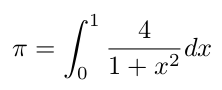You are here:   ArielOrtiz.com > Programming Languages > Computing Pi# Computing Pi

Numerical integration is a method of computing an approximation of the area under the curve of a function, especially when the exact integral cannot be solved. For example, the value of the constant pi can be defined by the following integral. However, rather than solve this integral exactly, we can approximate the solution by use of numerical integration:The following C code is an implementation of the numerical integration midpoint rectangle rule to solve the integral just shown. To compute an approximation of the area under the curve, we must compute the area of some number of rectangles (`num_rects`) by finding the midpoint (`mid`) of each rectangle and computing the height of that rectangle (`height`), which is simply the function value at that midpoint. We add together the heights of all the rectangles (`sum`) and, once computed, we multiply the sum of the heights by the width of the rectangles (`width`) to determine the desired approximation of the total area (`area`) and the value of pi.

```int main(void) {
long num_rects = 100000;
double mid, height, width, area;
double sum = 0.0;

width = 1.0 / (double) num_rects;
for (long i = 0; i < num_rects; i++) {
mid = (i + 0.5) * width;
height = 4.0 / (1.0 + mid * mid);
sum += height;
}
area = width * sum;
printf("Computed pi = %f\n", area);
return 0;
}
```

Reference: [BRESHEARS] pp. 31 & 32.